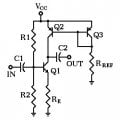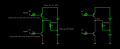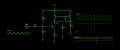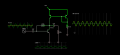# Understanding common emitter amplifier with active load

#### silv3r.m00n

Joined Apr 15, 2010
70

and the circuit isThe Rref and Q3 control the (max) quiescent current through the Q2 as a current mirror.
I guess the current through Q1 collector is manipulated by variations in the base voltage.

1. when base is HIGH, current through Q1 is HIGH, and no current is available for OUT. OUT = Current Sink / Negative Output.
2. when base is LOW, current through Q1 is LOW, and current is available for OUT. OUT = Current Source / Positive Output.

The output seems to be in the form of Current and NOT Voltage.

Is it possible to calculate the OUT voltage in this circuit and the gain ?
Or is it just not applicable in this case, until another stage converts the current output to voltage ?

Last edited:

#### Ian0

Joined Aug 7, 2020
8,387
It's not the most helpful article, is it?
So the output voltage is equal to the Q1 and Q2 collector current multiplied by the effective load impedance, and they give that as
"Now, the effective value of RL is ROUT of the mirror, which for this circuit is rO ≈ VA/IC"
IC is the current in Q1 and Q2 collectors, but what is Va? They don't tell you, but it is the "Early Voltage". And what's that?
If you get the graph from your transistor datasheet that looks like this, and extend the straight bit of each line to the left (ignoring the curved part next to the y-axis) you can just about imagine that all the lines would meet and cross the X axis at some point way off the page to the left. That point is the Early voltage, VA.
It's not a particularly accurate parameter, and it tends to be lower for pnp transistors than for npn transistors.
Actually you have two Ro values in parallel, one for each transistor. I wouldn't bother trying to work out what it is, because you'll most likely be wrong, just hope that they are large. To make sure that they are, choose transistors with the flattest possible curves.
The most important thing that determines the gain is actually missing from the diagram - it's the LOAD impedance - the input to the next stage or your measuring equipment or whatever comes next.
The load impedance is in parallel with the two Ro impedances, and if the load impedance is small compared to Ro, then the output impedance will be much the same as the load impedance, and the gain output voltage will be the Q1|Q2 collector current multiplied by the load impedance.
Now when it says the Q1|Q2 collector current, it means the AC current, not the DC current. And the AC current is the AC signal voltage, divided by RE (or, more accurately, Re+RE, where Re is the transistor's internal emitter resistance.
Re is kT/Q divided by emitter current. k=Boltzman's constant, T=absolute temperature and Q = charge on the electron.
kT/Q = 25mV, and this time it IS the DC current.

#### crutschow

Joined Mar 14, 2008
32,899
That circuit won't work in practice since the collector current of Q1 as determined by R1, R2, and Re, must exactly match the current as generated by the current-mirror consisting of Q2, Q3, and Rref.
There will always be component tolerances to cause one current to be slightly higher than the other, and the Q1 will either saturate or go to near the positive rail.

For that circuit to work you would need negative DC feedback (while blocking any AC feedback) from Q1's collector voltage to control the current-mirrors current.

•ronsimpson and Ian0

#### silv3r.m00n

Joined Apr 15, 2010
70
That circuit won't work in practice since the collector current of Q1 as determined by R1, R2, and Re, must exactly match the current as generated by the current-mirror consisting of Q2, Q3, and Rref.
There will always be component tolerances to cause one current to be slightly higher than the other, and the Q1 will either saturate or go to near the positive rail.

For that circuit to work you would need negative DC feedback (while blocking any AC feedback) from Q1's collector voltage to control the current-mirrors current.
1. my guess is - the currents through Q1 and Q2 do not need to match.

If Q1 is "MORE OPEN" than Q2 then OUT will act as current SINK = negative output.
If Q2 is "MORE OPEN" than Q1 then OUT will act as current SOURCE = positive output.

I created a small falstad simulation to try this:
https://tinyurl.com/y3h6vws3

ScreenshotThe current through R(load) is reverse in 2nd circuit.

In both circuits, one transistor is more open than the other.

The MORE open transistor is always SATURATED (not getting enough collector current) ...
whereas the LESS open transistor is ACTIVE ( having ample collector current).

So the same thing should happen in the active load amplifier circuit, posted earlier:I was actually reading Opamp internal circuit and its working. The differential stage of opamps like 741 actually use ACTIVE COLLECTOR LOADS that mirror currents from a reference, like shown here:

So the approach of active collector load should be working indeed.

Last edited:

#### crutschow

Joined Mar 14, 2008
32,899
The less open transistor is always SATURATED
Then it's not active.
Both transistors would have to be in the active region for the circuit to properly operate.

The 741 circuit is not the same as you have.
Both the emitter and collector have current-mirror control.

#### silv3r.m00n

Joined Apr 15, 2010
70
Then it's not active.
Both transistors would have to be in the active region for the circuit to properly operate.

The 741 circuit is not the same as you have.
Both the emitter and collector have current-mirror control.
I fiddled with the circuit and found some values, where both transistors are ACTIVE.Getting some amplification.
input: 100 mV
v(o): 450 mV

This time OUT is always current source and varies in the +ve region.

I do feel there are some drawbacks in the circuit, but not able to figure out.
Could you explain with some example.

#### crutschow

Joined Mar 14, 2008
32,899
I do feel there are some drawbacks in the circuit, but not able to figure out.
Could you explain with some example.
The problem is what I stated in post #3.
Since the collector currents of Q1 and Q2 have to exactly match for Q1 to stay in its active region, and that's not possible in a real circuit due to component tolerances and temperature drift.
The example of that is the circuit you have.

#### Ian0

Joined Aug 7, 2020
8,387
You’re making it with SPICE transistors. Try making it with real ones!
as Crutschow said earlier, it depends on the transistors having EXACTLY the same parameters, and remaining exactly matched as the temperature changes.
This circuit DOES work, but ONLY if there is a DC feedback loop around it somewhere to keep things in balance.
That’s why it is most often seen with a long-tailed pair, where the currents balance out.
Look up “long tailed pair with current mirror load”

#### Danko

Joined Nov 22, 2017
1,735

#### Attachments

• 2.1 KB Views: 3
Last edited:

#### silv3r.m00n

Joined Apr 15, 2010
70
That circuit won't work in practice since the collector current of Q1 as determined by R1, R2, and Re, must exactly match the current as generated by the current-mirror consisting of Q2, Q3, and Rref.
There will always be component tolerances to cause one current to be slightly higher than the other, and the Q1 will either saturate or go to near the positive rail.

For that circuit to work you would need negative DC feedback (while blocking any AC feedback) from Q1's collector voltage to control the current-mirrors current.
Found another circuit with active load that seems to work better but not sure if its correct.
Gain is around 100. (10mV -> 1 V).The collector base bias resistor keeps both transistors (amplifier and load) in active region.
it also keeps collector dc point at half of supply voltage.

Notes:

#### silv3r.m00n

Joined Apr 15, 2010
70
Here is an example of a dynamic load. Voltage amplification is performed by the first stage!View attachment 223241
thanks for sharing this circuit, its very interesting.
to me it appears less like active load and more like a positive feedback system.

Isn't C7+Q3 acting like positive feedback to the amplifier stage ?
also is it possible to derive the expression for the final gain ?

#### crutschow

Joined Mar 14, 2008
32,899
Found another circuit with active load
For proper operation I believe that circuit should have the collector-base short on the left transistor not the right.
As shown the output has essentially no gain.

#### silv3r.m00n

Joined Apr 15, 2010
70
For proper operation I believe that circuit should have the collector-base short on the left transistor not the right.
As shown the output has essentially no gain.
1. you mean the top left transistor ? but the top part is supposed to be a current mirror controlled by the right transistor.
i guess you are referring to the setup of a active load differential amplifier.

2. the output V(o) is about 1000 mV whereas the input is only 10 mV.
how come there is no gain ?

#### Bordodynov

Joined May 20, 2015
3,089
You can call the scheme I have shown using positive feedback, but this does not change the essence. It is a dynamic load. A dynamic load is a load that is greater in AC than in DC. For example a choke is also a dynamic load (especially at high frequencies. The advantage of the diagram shown is that the load of the first stage is high and the second transistor is included as a repeater. And the repeater has a higher resistance than the load. Yes, you can calculate the gain analytically (and I did it a long time ago, but I did it when I did not have a spice). I have now calculated the gain using the "measure" directives. I have placed the result of the gain of the first, normal and dynamic load amplification on the diagram as a comment (in blue). Gain calculation is cumbersome and I'm not sure if it's useful with Spice.